# HashTable in Java Example | Java HashTable Tutorial

HashTable in Java is a type of Collections framework which is used to store and manipulate the group of objects. It is an array of the list in java (known as a bucket) which is used to implement a hash table, which maps keys to values. Hashtable contains unique elements. The initial default capacity of the Hashtable class is 11and doesn’t allow null keys and values.

## HashTable in Java

Java Hashtable class implements the hashtable, which maps keys to the values. It inherits the Dictionary class and implements the Map interface. If we want to store and retrieve objects from a hashtable successfully, the objects used as keys must implement a hashCode method and the equals method.

1. It is similar to the HashMap, but it is synchronized.
2. Hashtable stores key/value pair in the hash table.
3. In the Hashtable, we specify the object that is used as a key, and the value we want to associate to that key. The key is then hashed, and it resulting the hash code is used as an index at which a value is stored within the table.

## #Key Points of Java Hashtable class

1. A Hashtable is the array of a list. Each list is known as the bucket. The position of the bucket is identified by calling a hashcode() method. The Hashtable contains values based on the key.
2. Java Hashtable class contains unique items.
3. Java Hashtable class doesn’t allow the null key or value.
4. Java Hashtable class is synchronized.
5. The initial by default capacity of a Hashtable class is 11 whereas the loadFactor is 0.75.

## #Working and storage of data in Hashtable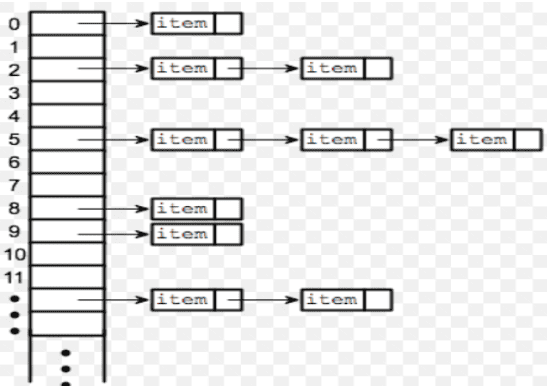In Hashtable, we specify the object that is used as a key, and the value we want to associate to that key.

## #Hashtable class declaration

Let’s understand the declaration of Hashtable in java.util.Hashtable class.

`public class Hashtable<K,V> extends Dictionary<K,V> implements Map<K,V>, Cloneable, Serializable`

The Hashtable class extends Dictionary class and implements Maps, Cloneable and Serializable interface.

Here,

1. ‘K’ is a type of Key.
2. ‘V’ is a type of mapped values to keys.

## #When to use Hashtable

Hashtable can be used in many different contexts. See the using below examples.

#Search

Hashing is useful for indexing large storage spaces so that you can look things up in them without reading their entire contents every time.

e.g., In Dictionary, Words will be the keys in a Hashtable, as they are supposed to be unique. Definitions, on the other hand, will be the values according to the keys or words from the dictionary.

#Programming languages

Hash tables are a convenient way to implement the mechanism that connects a variable’s name to its memory location, e.g., for storing any data and value according to their data types in the memory.

## #Constructors provided by the HashTable class

Hashtable class has four constructors, which are the following.

1. Hashtable (): It is the default constructor of a hash table it instantiates the Hashtable class.
2. Hashtable (int size): It creates a hash table that has an initial size specified by size.
3. Hashtable (int size, float fillRatio): It constructs the new, empty HashTable of specified initial size and fills ratio.
4. Hashtable (Map m): It constructs the HashTable that is initialized with the key-value pairs in the specified map.

Fill ratio determines how the full HashTable can be before its capacity is increased. Its Value lies between 0.0 to 1.0.

## #Methods provided by the HashTable class

The methods in the HashTable class are very similar to HashMap.

### #Object put (Object key, Object value)

It maps a specified key to a specified value in the HashTable. Neither a key nor the value can be null. See the following program.

```import java.util.Hashtable;
import java.util.Iterator;

public class p {
public static void main(String[] args) {

Hashtable<Integer, String> h1 = new Hashtable<>();// Created Hashtable

h1.put(1, "H");
h1.put(2, "A");
h1.put(3, "S");
h1.put(4, "H");
h1.put(5, "T");
h1.put(6, "A");
h1.put(7, "B");
h1.put(8, "L");
h1.put(9, "E");

System.out.println(h1);
}
}```

See the following output.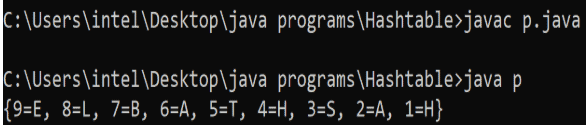### #Object get (Object key)

It returns a value to which the specified key is mapped. Returns the null if no such key is found.

See the following program.

```import java.util.Hashtable;

public class g {
public static void main(String[] args) {

Hashtable<Integer, String> h = new Hashtable<>();// Created Hashtable

h.put(1, "H");
h.put(2, "A");
h.put(3, "S");
h.put(4, "H");
h.put(5, "T");
h.put(6, "A");
h.put(7, "B");
h.put(8, "L");
h.put(9, "E");

System.out.println(h);

// Get a mapping by key
String v = h.get(4); // to get the data at 4th index
System.out.println(v); // to print the value at above index

}
}```

See the following output.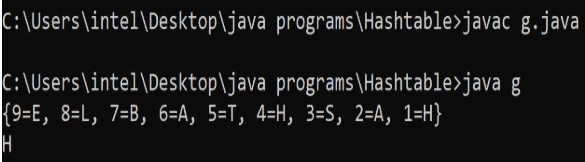### #Object remove (Object key)

It removes a key (and its corresponding value) from the HashTable. See the following file.

```import java.util.Hashtable;
import java.util.Iterator;

public class r {
public static void main(String[] args) {

Hashtable<Integer, String> h = new Hashtable<>();// Created Hashtable

h.put(1, "H");
h.put(2, "A");
h.put(3, "S");
h.put(4, "H");
h.put(5, "T");
h.put(6, "A");
h.put(7, "B");
h.put(8, "L");
h.put(9, "E");

System.out.println(h);

// Remove a mapping
h.remove(5); // 5 is deleted
System.out.println(h);
}
}```

See the output.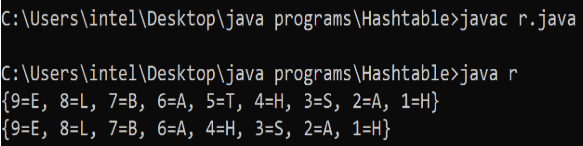### #boolean contains (Object value)

It returns true if the specified value exists within the hash table for any pair, else return false. Note that the boolean contains() method is identical in functionality to containsValue() function.

See the following program.

```import java.util.Hashtable;

public class c1 {
public static void main(String[] args) {

Hashtable<Integer, String> h = new Hashtable<>();// Created Hashtable

h.put(1, "H");
h.put(2, "A");
h.put(3, "S");
h.put(4, "H");
h.put(5, "T");
h.put(6, "A");
h.put(7, "B");
h.put(8, "L");
h.put(9, "E");

System.out.println(h);

// checking L is present in the table or not
System.out.println("Is the value 'L' present? " + h.contains("L"));
}
}```

See the output.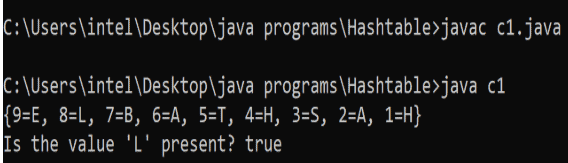### #boolean containsValue(Object value)

It returns true if the specified value exists within the hash table for any pair, else return false.

```import java.util.Hashtable;

public class c2 {
public static void main(String[] args) {

Hashtable<Integer, String> h = new Hashtable<>();// Created Hashtable

h.put(1, "H");
h.put(2, "A");
h.put(3, "S");
h.put(4, "H");
h.put(5, "T");
h.put(6, "A");
h.put(7, "B");
h.put(8, "L");
h.put(9, "E");

System.out.println(h);

// check whether a value exists or not
if (h.containsValue("S"))
System.out.println("value found in table");

}
}```

See the following output.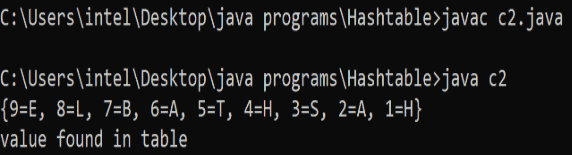### #boolean containsKey (Object key)

It returns true if the specified key exists within the hash table for any pair, else return false. See the following code.

```import java.util.Hashtable;
import java.util.Iterator;

public class c3 {
public static void main(String[] args) {

Hashtable<Integer, String> h = new Hashtable<>();// Created Hashtable

h.put(1, "H");
h.put(2, "A");
h.put(3, "S");
h.put(4, "H");
h.put(5, "T");
h.put(6, "A");
h.put(7, "B");
h.put(8, "L");
h.put(9, "E");

System.out.println(h);

// check whether a key exists or not
if (h.containsKey(9))
System.out.println("Key found in table");

}
}```

See the output.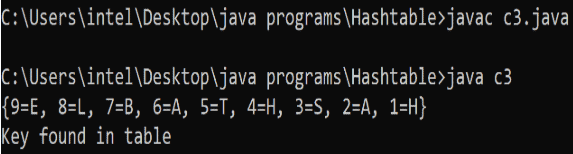### #boolean isEmpty()

It returns true if a Hashtable is empty; returns false if it contains at least one key. See the following code.

```import java.util.Hashtable;
import java.util.Iterator;

public class e {
public static void main(String[] args) {

Hashtable<Integer, String> h = new Hashtable<>();// Created Hashtable

h.put(1, "H");
h.put(2, "A");
h.put(3, "S");
h.put(4, "H");
h.put(5, "T");
h.put(6, "A");
h.put(7, "B");
h.put(8, "L");
h.put(9, "E");

System.out.println(h);
// clear hash table h
h.clear();

// checking whether hash table h is empty or not
if (h.isEmpty())
System.out.println("yes hash table is empty");

}
}```

See the following output.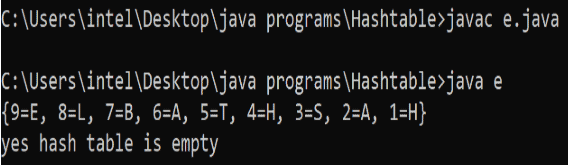### #void clear()

It is used to remove all pairs in the HashTable. See the following code.

```import java.util.Iterator;

public class c {
public static void main(String[] args) {

Hashtable<Integer, String> h = new Hashtable<>();// Created Hashtable

h.put(1, "H");
h.put(2, "A");
h.put(3, "S");
h.put(4, "H");
h.put(5, "T");
h.put(6, "A");
h.put(7, "B");
h.put(8, "L");
h.put(9, "E");

System.out.println(h);
// clear hash table h
h.clear();
System.out.println(h);

}
}```

This output will be not available because data is cleared.

### #entrySet()

It is used to get a set view of the entries contained in this hash table. See the following code.

```// Java code illustrating entreysent() method
import java.util.*;
class es {
public static void main(String[] arg)
{
// creating a hash table
Hashtable<Integer, String> h =
new Hashtable<Integer, String>();

h.put(3, "C");
h.put(2, "B");
h.put(1, "A");

// creating set view for hash table
Set s = h.entrySet();

// printing set entries
System.out.println("set entries: " + s);
}
}```

See the output.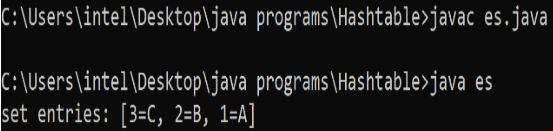### #String toString()

Returns a string equivalent of a hash table. See the following code.

```// Java code illustrating toString() method
import java.util.*;
class s {
public static void main(String[] arg)
{
// creating a hash table
Hashtable<Integer, String> h =
new Hashtable<Integer, String>();

h.put(3, "A");
h.put(2, "B");
h.put(1, "C");

// String equivalent of map
System.out.println("string equivalent" +
" of map: " + h.toString());
}
}```

See the following output.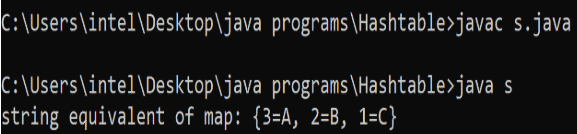### #int size()

The size() method returns the number of entries in the hash table. See the program.

```// Java code to understand size() method
import java.util.*;

class size1 {
public static void main(String[] arg) {
// creating a hash table
Hashtable<String, Integer> m = new Hashtable<String, Integer>();

// enter name/m pair
m.put("A", new Integer(345));
m.put("B", new Integer(245));
m.put("C", new Integer(790));
m.put("D", new Integer(365));

// size of hash table
System.out.println("Size is: " + m.size());
}
}```

See the following output.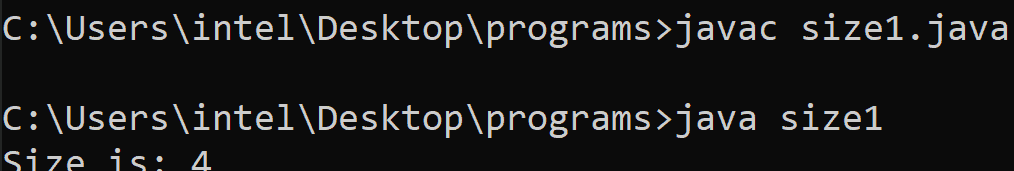### #KeySet()

The KeySet() method is used to get the Set view of all the keys contained in a particular hash table.

```// Java code to understand keySet() method
import java.util.*;

class keyset1 {
public static void main(String[] arg) {
// creating a hash table
Hashtable<Integer, String> h = new Hashtable<Integer, String>();

h.put(3, "X");
h.put(2, "Y");
h.put(1, "Z");

// creating set view for keys
Set skey = h.keySet();

// checking key set
System.out.println("key set: " + skey);
}
}```

See the following output.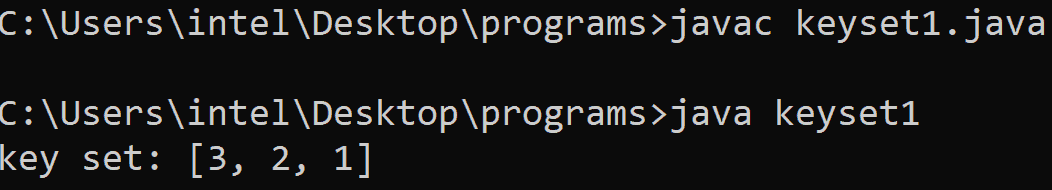### #int hashCode()

The hashCode() method returns the hash code value for this Map as per the definition in the Map interface. See the following code.

```// Java code to understand hashCode() method
import java.util.*;

class hashcode1 {
public static void main(String[] arg) {
// creating a hash table
Hashtable<Integer, String> h = new Hashtable<Integer, String>();

h.put(3, "three");
h.put(2, "two");
h.put(1, "one");

// obtaining hash code
System.out.println("hash code is: " + h.hashCode());
}
}```

See the following output.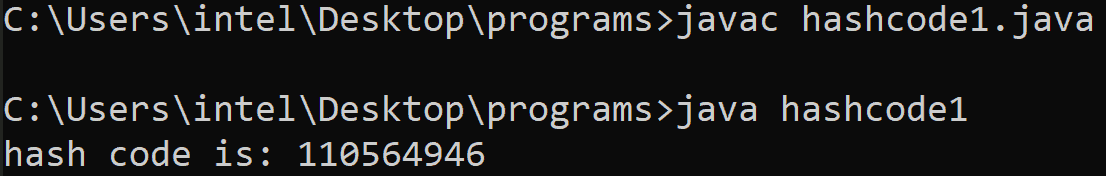### #computeIfAbsent()

The computeIfAbsent(Key, Function) method of HashMap class is used to compute the value for a given key using the given mapping function if the key is not already associated with a value (or is mapped to null) and enter that computed value in HashTable else null.

See the following program.

```// Java program to understand computeIfAbsent(Key, Function) method.
import java.util.*;

public class computeifabsent {

// Main method
public static void main(String[] args) {

// create a table and add some values
Map<String, Integer> T = new Hashtable<>();
T.put("X", 10);
T.put("Y", 20);
T.put("Z", 30);

// print map details
System.out.println("hashTable: " + T.toString());

T.computeIfAbsent("newX", k -> 100); // provide value for new key which is absent using computeIfAbsent method
T.computeIfAbsent("newY", k -> 200);

System.out.println("new hashTable" + T); // print new mapping
}
}```

See the following output.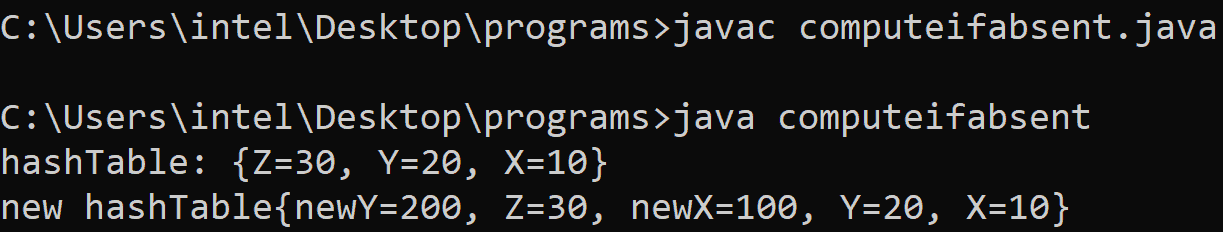### #putIfAbsent()

The putIfAbsent() method of Hashtable class which allows mapping a value to a given key if a given key is not associated with the value or mapped to null. See the program.

```// Java program to understand putIfAbsent(key, value) method.

import java.util.*;

public class putifabsent1 {

// Main method
public static void main(String[] args) {

// create a table and add some values
Map<String, Integer> t = new Hashtable<>();

t.put("A", 100);
t.put("B", 200);
t.put("C", 300);

// print map details
System.out.println("hashTable: " + t.toString());

// Inserting non-existing key with value
// using putIfAbsent method
String retValue = String.valueOf(t.putIfAbsent("E", 400));

// Print the returned value
System.out.println("Returned value " + "for Key 'E' is: " + retValue);

// print new mapping
System.out.println("hashTable: " + t);

// Inserting existing key with value
// using putIfAbsent method
retValue = String.valueOf(t.putIfAbsent("Book", 4500));

// Print the returned value
System.out.println("Returned value" + " for key 'B' is: " + retValue);

// print new mapping
System.out.println("hashTable: " + t);
}
}```

See the following output.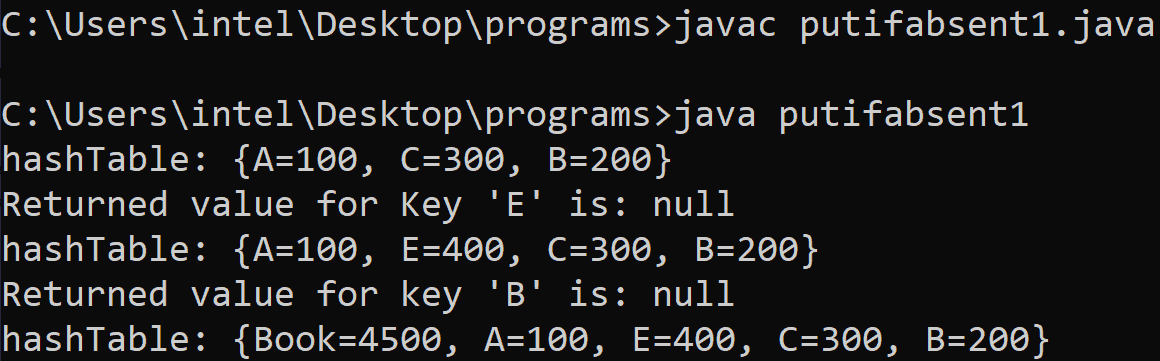### #enumeration keys()

The enumeration keys() method is used to get an enumeration of the keys contained in the hashtable. See the program.

```// Java code illustrating keys() method
import java.util.*;

class enumkeys {
public static void main(String[] arg) {
// creating a hash table
Hashtable<Integer, String> h = new Hashtable<Integer, String>();

Hashtable<Integer, String> h1 = new Hashtable<Integer, String>();

h.put(3, "three");
h.put(2, "two");
h.put(1, "one");

// create enumeration

Enumeration e1 = h.keys();
System.out.println("display key:");

while (e1.hasMoreElements()) {
System.out.println(e1.nextElement());
}
}
}```

See the output.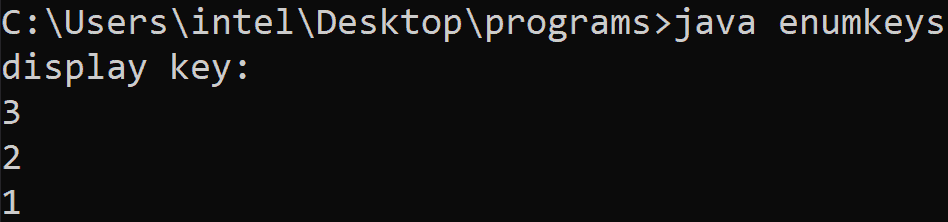### #values()

The values() method is used to check the values of hashTable. See the following code.

```// Java code to understand values() method
import java.util.*;

class value1 {
public static void main(String[] arg) {
// creating a hash table
Hashtable<Integer, String> h = new Hashtable<Integer, String>();

h.put(3, "X");
h.put(2, "Y");
h.put(1, "Z");

// creating set view for hash table
Set s = h.entrySet();

// checking collection view of values
System.out.println("collection values: " + h.values());
}
}```

See the following output.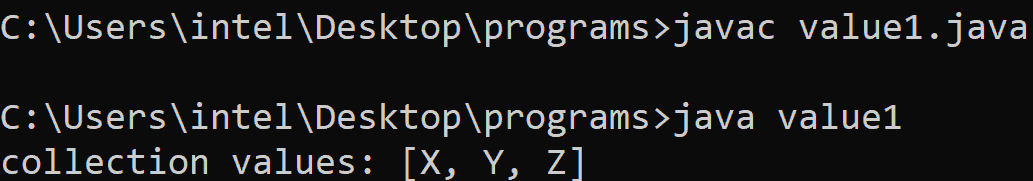Finally, HashTable in Java Example is over.

This site uses Akismet to reduce spam. Learn how your comment data is processed.